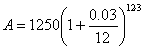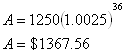Formula
Example 1

You are investing \$1250 into an account that yields 3%, compounded monthly, and you plan to invest for a total of 3 years. How much money will you have at the end of the three years?

Step 1. Identify the variables.

A is the ending amount, this is what you are trying to determine.

P is the beginning amount, in this case \$1250.

r is the interest rate written in the form of a decimal, in this case 0.03.

t is the time in years, in this case 3 years.

n is the number of times compounded per year, in this case it is being compounded monthly and therefore n = 12.

Step 2. Substitute the variables into the formula.Step 3. Calculate the formula, you may need a calculator.